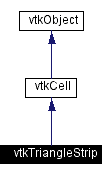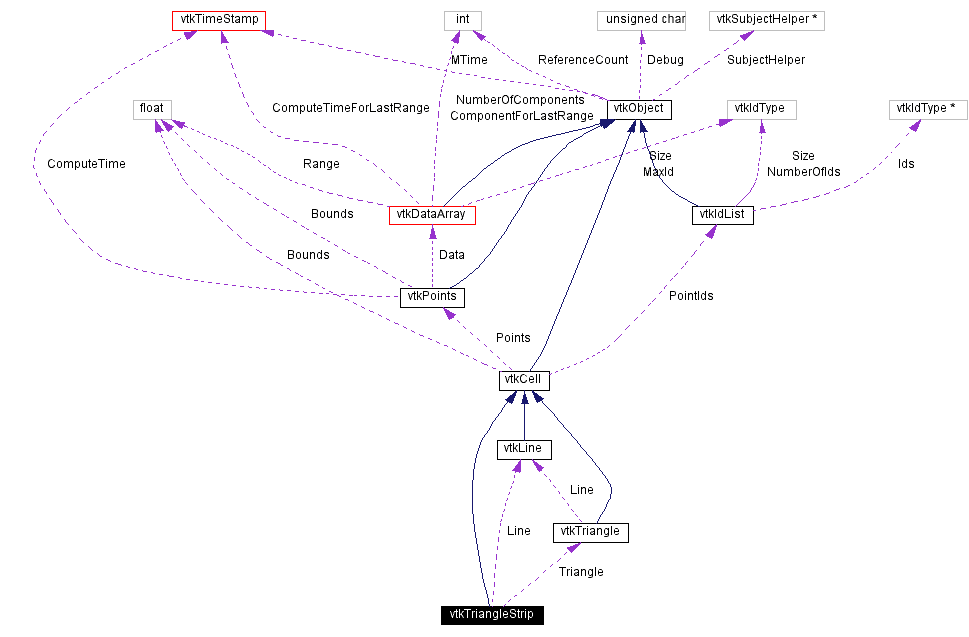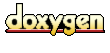Main Page   Class Hierarchy   Alphabetical List   Compound List   File List   Compound Members   File Members   Related Pages

# vtkTriangleStrip Class Reference

a cell that represents a triangle strip. More...

`#include <vtkTriangleStrip.h>`

Inheritance diagram for vtkTriangleStrip:[legend]
Collaboration diagram for vtkTriangleStrip:[legend]
List of all members.

## Public Methods

virtual const char * GetClassName ()
virtual int IsA (const char *type)
int EvaluatePosition (float x, float *closestPoint, int &subId, float pcoords, float &dist2, float *weights)
void EvaluateLocation (int &subId, float pcoords, float x, float *weights)
int IntersectWithLine (float p1, float p2, float tol, float &t, float x, float pcoords, int &subId)
int Triangulate (int index, vtkIdList *ptIds, vtkPoints *pts)
void Derivatives (int subId, float pcoords, float *values, int dim, float *derivs)
int GetParametricCenter (float pcoords)
vtkCellMakeObject ()
int GetCellType ()
int GetCellDimension ()
int GetNumberOfEdges ()
int GetNumberOfFaces ()
vtkCellGetEdge (int edgeId)
vtkCellGetFace (int vtkNotUsed(faceId))
int CellBoundary (int subId, float pcoords, vtkIdList *pts)
void Contour (float value, vtkDataArray *cellScalars, vtkPointLocator *locator, vtkCellArray *verts, vtkCellArray *lines, vtkCellArray *polys, vtkPointData *inPd, vtkPointData *outPd, vtkCellData *inCd, vtkIdType cellId, vtkCellData *outCd)
void Clip (float value, vtkDataArray *cellScalars, vtkPointLocator *locator, vtkCellArray *polys, vtkPointData *inPd, vtkPointData *outPd, vtkCellData *inCd, vtkIdType cellId, vtkCellData *outCd, int insideOut)

## Static Public Methods

vtkTriangleStrip * New ()
int IsTypeOf (const char *type)
vtkTriangleStrip * SafeDownCast (vtkObject *o)
void DecomposeStrip (int npts, vtkIdType *pts, vtkCellArray *tris)

## Protected Methods

vtkTriangleStrip ()
~vtkTriangleStrip ()

## Protected Attributes

vtkLineLine
vtkTriangleTriangle

## Detailed Description

a cell that represents a triangle strip.

Date:
2001/11/02 16:41:23
Revision:
1.59

vtkTriangleStrip is a concrete implementation of vtkCell to represent a 2D triangle strip. A triangle strip is a compact representation of triangles connected edge to edge in strip fashion. The connectivity of a triangle strip is three points defining an initial triangle, then for each additional triangle, a single point that, combined with the previous two points, defines the next triangle.

Examples:
vtkTriangleStrip (Examples)
Tests:
vtkTriangleStrip (Tests)

Definition at line 67 of file vtkTriangleStrip.h.

## Constructor & Destructor Documentation

 vtkTriangleStrip::vtkTriangleStrip ( ) ` [protected]`

 vtkTriangleStrip::~vtkTriangleStrip ( ) ` [protected]`

## Member Function Documentation

 vtkTriangleStrip* vtkTriangleStrip::New ( ) ` [static]`
 Create an object with Debug turned off, modified time initialized to zero, and reference counting on. Reimplemented from vtkObject.

 virtual const char* vtkTriangleStrip::GetClassName ( ) ` [virtual]`
 Return the class name as a string. This method is defined in all subclasses of vtkObject with the vtkTypeMacro found in vtkSetGet.h. Reimplemented from vtkCell.

 int vtkTriangleStrip::IsTypeOf ( const char * type ) ` [static]`
 Return 1 if this class type is the same type of (or a subclass of) the named class. Returns 0 otherwise. This method works in combination with vtkTypeMacro found in vtkSetGet.h. Reimplemented from vtkCell.

 virtual int vtkTriangleStrip::IsA ( const char * type ) ` [virtual]`
 Return 1 if this class is the same type of (or a subclass of) the named class. Returns 0 otherwise. This method works in combination with vtkTypeMacro found in vtkSetGet.h. Reimplemented from vtkCell.

 vtkTriangleStrip* vtkTriangleStrip::SafeDownCast ( vtkObject * o ) ` [static]`
 Will cast the supplied object to vtkObject* is this is a safe operation (i.e., a safe downcast); otherwise NULL is returned. This method is defined in all subclasses of vtkObject with the vtkTypeMacro found in vtkSetGet.h. Reimplemented from vtkCell.

 vtkCell* vtkTriangleStrip::MakeObject ( ) ` [virtual]`
 See the vtkCell API for descriptions of these methods. Reimplemented from vtkCell.

 int vtkTriangleStrip::GetCellType ( ) ` [inline, virtual]`
 See the vtkCell API for descriptions of these methods. Reimplemented from vtkCell. Definition at line 76 of file vtkTriangleStrip.h.

 int vtkTriangleStrip::GetCellDimension ( ) ` [inline, virtual]`
 See the vtkCell API for descriptions of these methods. Reimplemented from vtkCell. Definition at line 77 of file vtkTriangleStrip.h.

 int vtkTriangleStrip::GetNumberOfEdges ( ) ` [inline, virtual]`
 See the vtkCell API for descriptions of these methods. Reimplemented from vtkCell. Definition at line 78 of file vtkTriangleStrip.h.

 int vtkTriangleStrip::GetNumberOfFaces ( ) ` [inline, virtual]`
 See the vtkCell API for descriptions of these methods. Reimplemented from vtkCell. Definition at line 79 of file vtkTriangleStrip.h.

 vtkCell* vtkTriangleStrip::GetEdge ( int edgeId ) ` [virtual]`
 See the vtkCell API for descriptions of these methods. Reimplemented from vtkCell.

 vtkCell* vtkTriangleStrip::GetFace ( int vtkNotUsed(faceId) ) ` [inline]`
 See the vtkCell API for descriptions of these methods. Definition at line 81 of file vtkTriangleStrip.h.

 int vtkTriangleStrip::CellBoundary ( int subId, float pcoords, vtkIdList * pts ) ` [virtual]`
 See the vtkCell API for descriptions of these methods. Reimplemented from vtkCell.

 void vtkTriangleStrip::Contour ( float value, vtkDataArray * cellScalars, vtkPointLocator * locator, vtkCellArray * verts, vtkCellArray * lines, vtkCellArray * polys, vtkPointData * inPd, vtkPointData * outPd, vtkCellData * inCd, vtkIdType cellId, vtkCellData * outCd ) ` [virtual]`
 See the vtkCell API for descriptions of these methods. Reimplemented from vtkCell.

 void vtkTriangleStrip::Clip ( float value, vtkDataArray * cellScalars, vtkPointLocator * locator, vtkCellArray * polys, vtkPointData * inPd, vtkPointData * outPd, vtkCellData * inCd, vtkIdType cellId, vtkCellData * outCd, int insideOut ) ` [virtual]`
 See the vtkCell API for descriptions of these methods. Reimplemented from vtkCell.

 int vtkTriangleStrip::EvaluatePosition ( float x, float * closestPoint, int & subId, float pcoords, float & dist2, float * weights ) ` [virtual]`
 Given a point x return inside(=1) or outside(=0) cell; evaluate parametric coordinates, sub-cell id (!=0 only if cell is composite), distance squared of point x to cell (in particular, the sub-cell indicated), closest point on cell to x (unless closestPoint is null, in which case, the closest point and dist2 are not found), and interpolation weights in cell. (The number of weights is equal to the number of points defining the cell). Note: on rare occasions a -1 is returned from the method. This means that numerical error has occurred and all data returned from this method should be ignored. Also, inside/outside is determine parametrically. That is, a point is inside if it satisfies parametric limits. This can cause problems for cells of topological dimension 2 or less, since a point in 3D can project onto the cell within parametric limits but be "far" from the cell. Thus the value dist2 may be checked to determine true in/out. Reimplemented from vtkCell.

 void vtkTriangleStrip::EvaluateLocation ( int & subId, float pcoords, float x, float * weights ) ` [virtual]`
 Determine global coordinate (x) from subId and parametric coordinates. Also returns interpolation weights. (The number of weights is equal to the number of points in the cell.) Reimplemented from vtkCell.

 int vtkTriangleStrip::IntersectWithLine ( float p1, float p2, float tol, float & t, float x, float pcoords, int & subId ) ` [virtual]`
 Intersect with a ray. Return parametric coordinates (both line and cell) and global intersection coordinates, given ray definition and tolerance. The method returns non-zero value if intersection occurs. Reimplemented from vtkCell.

 int vtkTriangleStrip::Triangulate ( int index, vtkIdList * ptIds, vtkPoints * pts ) ` [virtual]`
 Generate simplices of proper dimension. If cell is 3D, tetrahedron are generated; if 2D triangles; if 1D lines; if 0D points. The form of the output is a sequence of points, each n+1 points (where n is topological cell dimension) defining a simplex. The index is a parameter that controls which triangulation to use (if more than one is possible). If numerical degeneracy encountered, 0 is returned, otherwise 1 is returned. Reimplemented from vtkCell.

 void vtkTriangleStrip::Derivatives ( int subId, float pcoords, float * values, int dim, float * derivs ) ` [virtual]`
 Compute derivatives given cell subId and parametric coordinates. The values array is a series of data value(s) at the cell points. There is a one-to-one correspondence between cell point and data value(s). Dim is the number of data values per cell point. Derivs are derivatives in the x-y-z coordinate directions for each data value. Thus, if computing derivatives for a scalar function in a hexahedron, dim=1, 8 values are supplied, and 3 deriv values are returned (i.e., derivatives in x-y-z directions). On the other hand, if computing derivatives of velocity (vx,vy,vz) dim=3, 24 values are supplied ((vx,vy,vz)1, (vx,vy,vz)2, ....()8), and 9 deriv values are returned ((d(vx)/dx),(d(vx)/dy),(d(vx)/dz), (d(vy)/dx),(d(vy)/dy), (d(vy)/dz), (d(vz)/dx),(d(vz)/dy),(d(vz)/dz)). Reimplemented from vtkCell.

 int vtkTriangleStrip::GetParametricCenter ( float pcoords ) ` [virtual]`
 Return the center of the point cloud in parametric coordinates. Reimplemented from vtkCell.

 void vtkTriangleStrip::DecomposeStrip ( int npts, vtkIdType * pts, vtkCellArray * tris ) ` [static]`
 Given a triangle strip, decompose it into a list of (triangle) polygons. The polygons are appended to the end of the list of triangles.

## Member Data Documentation

 vtkLine* vtkTriangleStrip::Line` [protected]`
 Definition at line 119 of file vtkTriangleStrip.h.

 vtkTriangle* vtkTriangleStrip::Triangle` [protected]`
 Definition at line 120 of file vtkTriangleStrip.h.

The documentation for this class was generated from the following file:

Generated on Thu Mar 28 14:44:40 2002 for VTK by1.2.11.1 written by Dimitri van Heesch, © 1997-2001